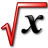TeX equation editor (Mathematical Formulas)
 Equation WYSIWYG Editor LaTex Viewer and Editor
 TeX Equation real-time Renderer HTML (Fast) Image Enable color Background color Text color Color Tools No transparent Background transparent Entire image transparent Height (0: AutoSize)This app is an equation editor for writing math equations, expressions, mathematical characters, and operations.
You can also generate an image of a mathematical formula using the TeX language (pronounced "tek" or "tech"). This is useful for displaying complex formulas on your web page.
TeX equation editor that creates graphical equations. Produces code for directly embedding equations into HTML websites, forums or blogs. Images may also be dragged into other applications like Word.

How to insert the image into a Google document
Copy the generated image to clipboard, and Paste it to Google documents (Ctrl+V, Apple Key+V).